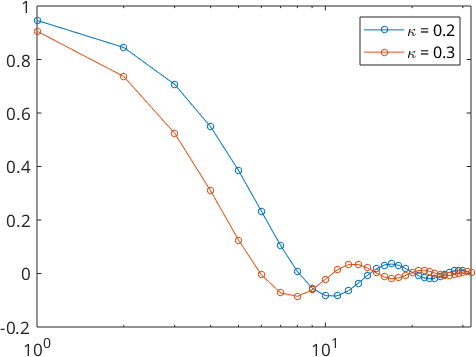SO(3)-Kernel Functions edit page

Also take a look at the page ODFShapes.

We examine some radial symmetric kernel functions $$\tilde \psi \colon \mathcal{SO}(3) \to \mathbb R$$ on $$\mathcal{SO}(3)$$. For rotations $${\bf R} \in \mathcal{SO}(3)$$ we write this $$\mathcal{SO}(3)$$-kernels as functions of $$t = \cos\frac{\omega ({\bf R})}2$$ on the real numbers. Hence we write

$\psi(t) = \tilde\psi ({\bf R}).$

Moreover, we have $$\psi \in L^2([-1,1],\sqrt{1-t^2}\mathrm{d}t)$$ and we describe these rotational kernel functions by there Chebyshev expansion

$\psi(t) = \sum\limits_{n=0}^{\infty} \hat\psi_n \, \mathcal U_{2n}(t)$

where $$\mathcal U_{n}$$ denotes the Chebyshev polynomials of second kind and degree $$n\in \mathbb N$$.

The class SO3Kernel is needed in MTEX to define the specific form of unimodal ODFs. It has to be passed as an argument when calling the methods uniformODF. Furthermore $$\mathcal{SO}(3)$$-Kernels are also used for computing an ODF from EBSD data.

Within the class SO3Kernel kernel functions are represented by their Chebyshev coefficients, that are stored in the field fun.A. As an example lets define an $$\mathcal{SO}(3)$$ kernel function with Chebyshev coefficients $$a_0 = 1$$, $$a_1 = 0$$, $$a_2 = 3$$ and $$a_3 = 1$$

We plot this function by evaluation of its Chebychev series in $$\cos(\frac{\omega}{2})$$ for $$\omega \in [-\pi,\pi]$$.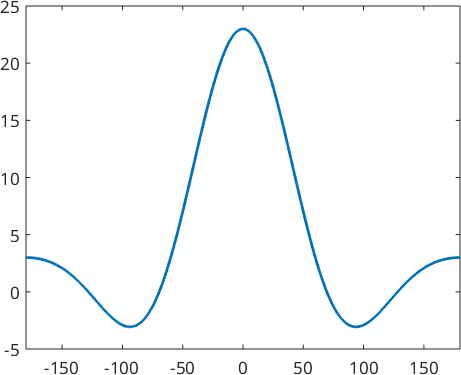We can define an SO3Fun from a kernel function $$\psi$$ at a specific orientation $$\bf R$$ by using the class SO3FunRBF, i.e.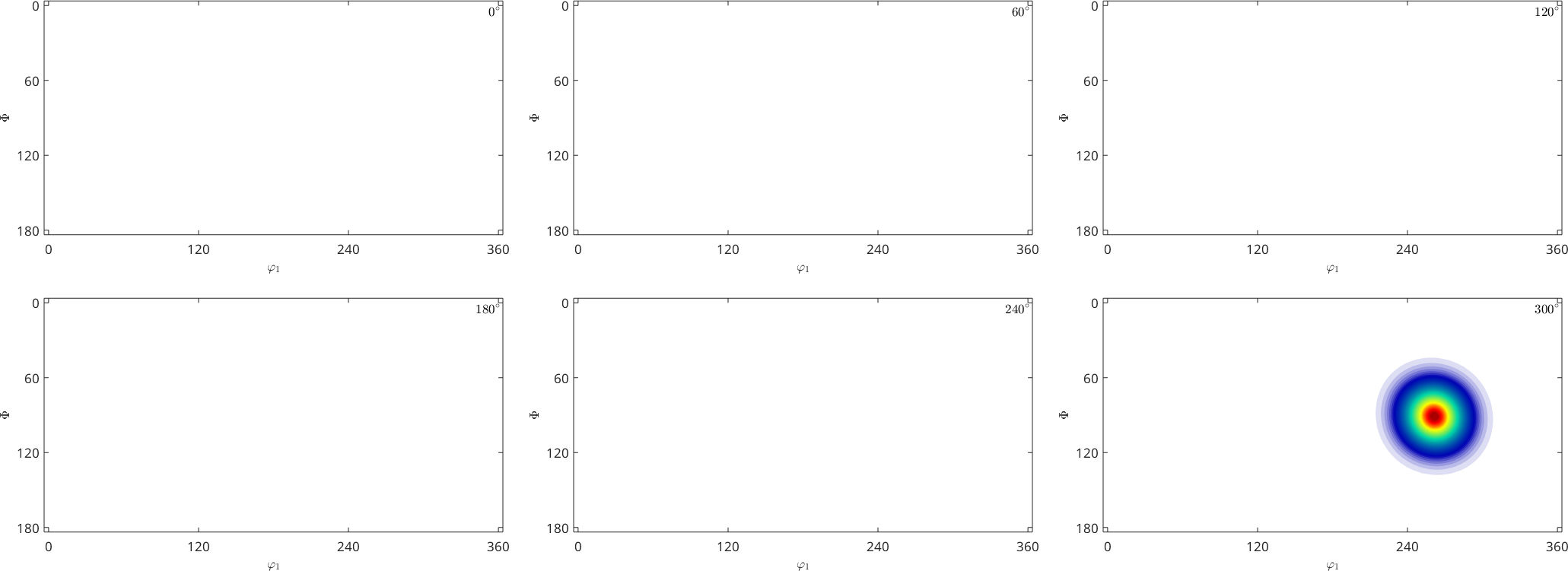The following kernel function are predefined in MTEX

A specific $$\mathcal{SO}(3)$$ kernel function like the de la Vallee Poussin kernel is specified by a half-width angle in orientation space ($$\mathcal{SO}(3)$$) or bandwidth in Fourier space, which is the maximum development in Fourier coefficients.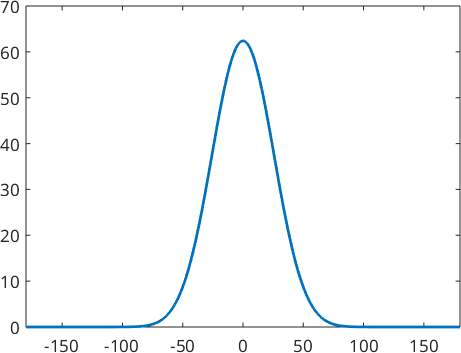In the following we want to look at some different types of $$\mathcal{SO}(3)$$ kernel functions.

## The de La Vallee Poussin Kernel

The de la Vallee Poussin kernel on $$\mathcal{SO}(3)$$ is defined by

$K(t) = \frac{B(\frac32,\frac12)}{B(\frac32,\kappa+\frac12)}\,t^{2\kappa}$

for $$t\in[0,1]$$, where $$B$$ denotes the Beta function. The de la Vallee Poussin kernel additionaly has the unique property that for a given halfwidth it can be described exactly by a finite number of Fourier coefficients. This kernel is recommended for Texture analysis as it is always positive in orientation space and there is no truncation error in Fourier space. Hence we can define the de la Vallee Poussin kernel $$\psi_{\kappa}$$ depending on a parameter $$\kappa \in \mathbb N \setminus \{0\}$$ by its finite Chebyshev expansion

$\psi_{\kappa}(t) = \frac{(\kappa+1)\,2^{2\kappa-1}}{\binom{2\kappa-1}{\kappa}} \, t^{2\kappa} = \binom{2\kappa+1}{\kappa}^{-1} \, \sum\limits_{n=0}^{\kappa} (2n+1)\,\binom{2\kappa+1}{\kappa-n} \, \mathcal U_{2n}(t).$

Lets construct two of them.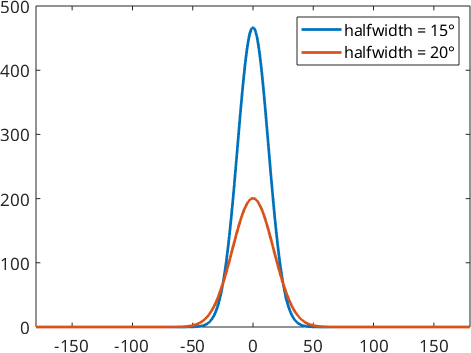Here the parameter $$\kappa$$ is $$40.34$$ for function $$\psi_1$$ and $$22.64$$ in function $$\psi_2$$.

We also take a look at the Fourier coefficients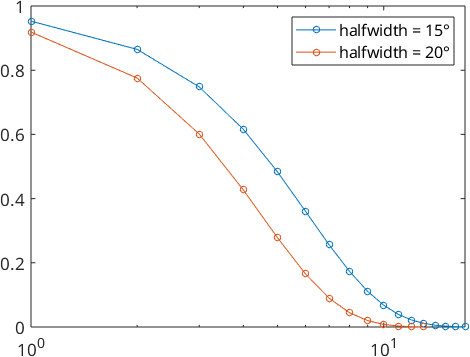## The Dirichlet Kernel

The Dirichlet kernel has the unique property of being a convergent finite series in Fourier coefficients with an integral of one. This kernel is recommended for calculating physical properties as the Fourier coefficients always have a value of one for a given bandwidth.

On the rotation group $$\mathcal{SO}(3)$$ the Dirichlet kernel $$\psi_N \in L^2(\mathcal{SO}(3))$$ is defined by its Chebyshev series

$\psi_N(t) = \sum\limits_{n=0}^N (2n+1) \, \mathcal U_{2n}(t).$

Lets construct two of them.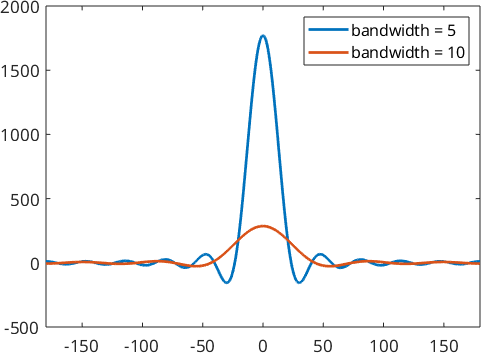By looking at the fourier coefficients we see, that they are exactly 1.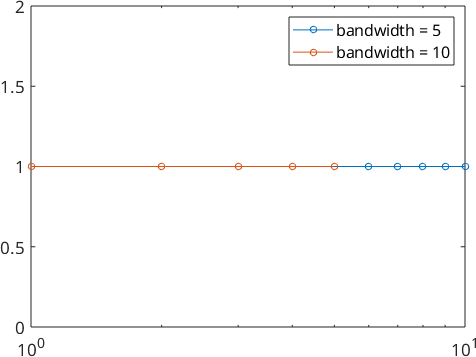## The Abel Poisson Kernel

The Abel Poisson kernel $$\psi_{\kappa}\in L^2(\mathcal{SO}(3))$$ is a nonnegative function depending on a parameter $$\kappa \in (0,1)$$ and is defined by its Chebyshev series

$\psi_{\kappa}(t) = \sum\limits_{n=0}^{\infty} (2n+1) \, \kappa^{2n} \, \mathcal U_{2n}(t).$

Lets construct two of them.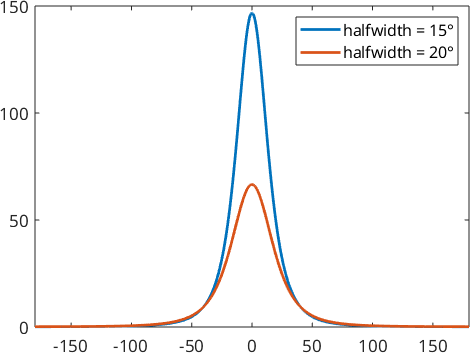Here the parameter $$\kappa$$ is $$0.82$$ for function $$\psi_1$$ and $$0.76$$ in function $$\psi_2$$.

We also take a look at the Fourier coefficients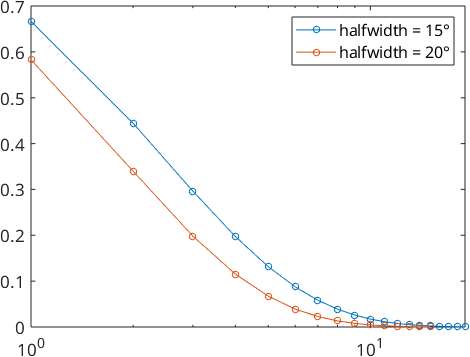## The von Mises Fisher Kernel

The von Mises Fisher kernel $$\psi_{\kappa}\in L^2(\mathcal{SO}(3))$$ is a nonnegative function depending on a parameter $$\kappa>0$$ and is defined by its Chebyshev series

$\psi_{\kappa}(t) = \sum_{n=0}^{\infty} \frac{\mathcal{I}_n(\kappa)-\mathcal{I}_{n+1}(\kappa)}{\mathcal{I}_0(\kappa)-\mathcal{I}_1(\kappa)} \, \mathcal U_{2n}(t)$

or directly by

$\psi_{\kappa}(\cos\frac{\omega({\bf R})}2) = \frac1{\mathcal{I}_0(\kappa)-\mathcal{I}_1(\kappa)} \, \mathrm{e}^{\kappa \cos\omega({\bf R})}$

while $$\mathcal I_n,\,n \in \mathbb N_0$$ denotes the the modified Bessel functions of first kind

$\mathcal I_n (\kappa) = \frac1{\pi} \int_0^{\pi} \mathrm e^{\kappa \, \cos \omega} \, \cos n\omega \, \mathrm d\omega.$

Lets construct two of this kernels.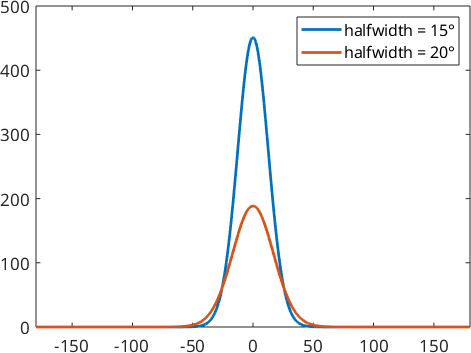Here the parameter $$\kappa$$ is $$20.34$$ for function $$\psi_1$$ and $$11.49$$ in function $$\psi_2$$.

We also take a look at the Fourier coefficients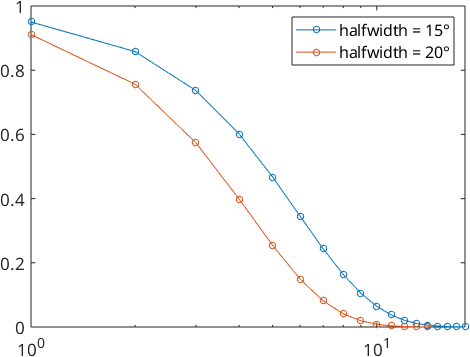## The Gauss Weierstrass Kernel

The Gauss Weierstrass kernel $$\psi_{\kappa}\in L^2(\mathcal{SO}(3))$$ is a nonnegative function depending on a parameter $$\kappa>0$$ and is defined by its Chebyshev series

$\psi_{\kappa}(t) = \sum\limits_{n=0}^{\infty} (2n+1) \, \mathrm e^{-n(n+1)\kappa} \, \mathcal U_{2n}(t).$

Lets construct two of them by the parameter $$\kappa$$.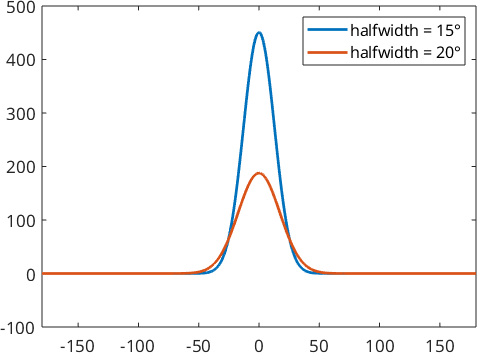We also take a look at the Fourier coefficients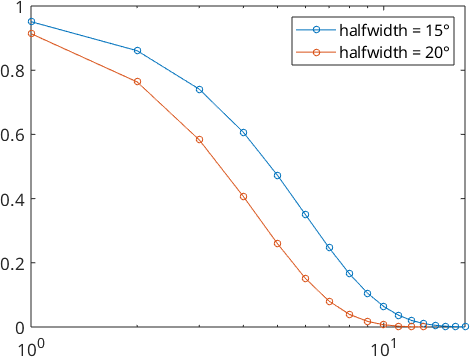## The Sobolev Kernel

The Sobolev kernel $$\psi_{s}\in L^2(\mathcal{SO}(3))$$ is a radial symmetric kernel function depending on a parameter $$s$$ and is defined by its Chebyshev series

$\psi_s(t) = \sum\limits_{n=0}^{\infty} (2n+1)\, (n(n+1))^s \, \mathcal U_{2n}(t).$

Lets construct two of them by the parameter $$s$$ and banwidth 15.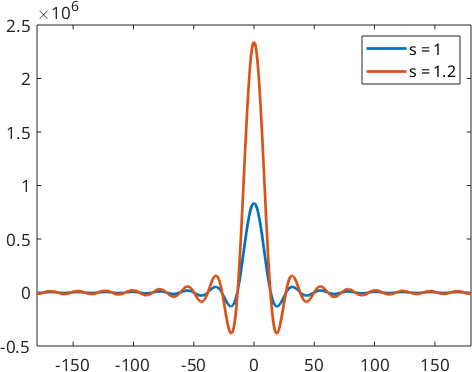We also take a look at the Fourier coefficients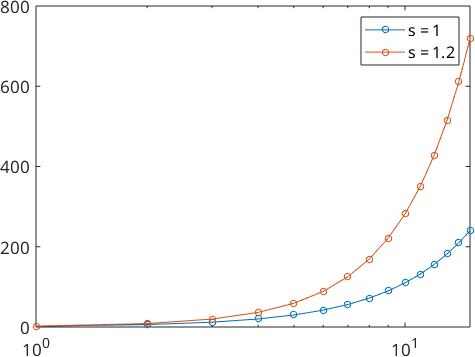## The Laplace Kernel

The Laplace kernel $$\psi\in L^2(\mathcal{SO}(3))$$ is a radial symmetric kernel function which is defined by its Chebyshev series

$\psi(t) = \sum\limits_{n=0}^{\infty} \frac{(2n+1)}{4\,n^2\,(2n+2)^2} \, \mathcal U_{2n}(t).$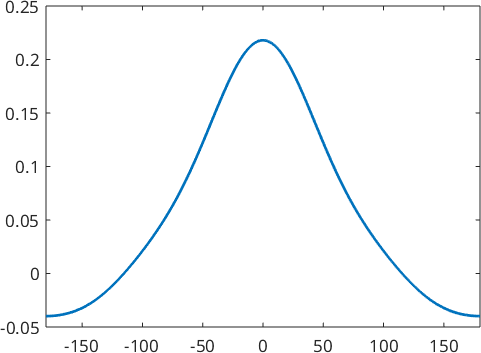We also take a look at the Fourier coefficients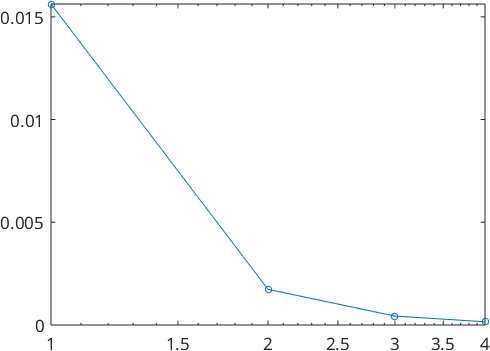## The Squared Singularity Kernel

The squared singularity kernel $$\psi_{\kappa}\in L^2(\mathcal{SO}(3))$$ is a nonnegative function depending on a parameter $$\kappa\in(0,1)$$ and is defined by its Chebyshev series

$\psi_{\kappa}(t) = \sum\limits_{n=0}^{\infty} \hat{f}_n(\kappa) \, \mathcal U_{2n}(t).$

where the chebychev coefficients follows a 3-term recurrsion

$$\hat{f}_0 = 1$$

$$\hat{f}_1 = \frac{1+\kappa^2}{2\kappa}-\frac1{\log\frac{1+\kappa}{1-\kappa}}$$

$$\hat{f}_n = \frac{(2n-3)(2n+1)(1+\kappa^2)}{(2n-1)(n-1)2\kappa} \, \hat{f}_{n-1}(\kappa)-\frac{2\kappa(n-2)(2n+1)}{2n-3} \, \hat{f}_{n-2}(\kappa)$$.

Lets construct two of them by the parameter $$\kappa$$.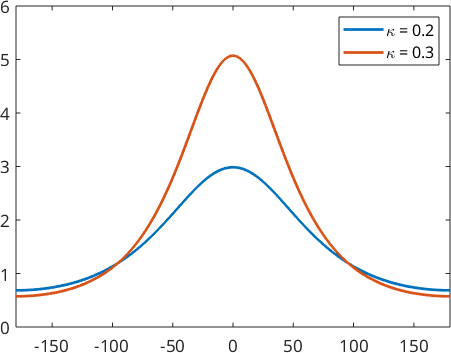We also take a look at the Fourier coefficients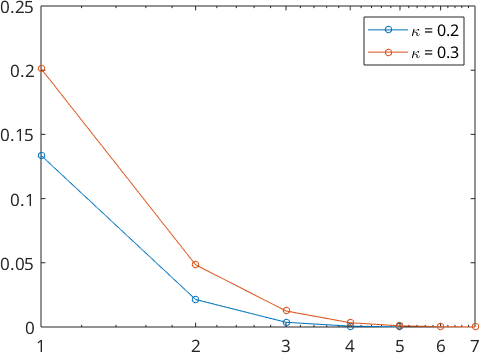## The Bump kernel

The bump kernel $$\tilde\psi_r\in L^2(\mathcal{SO}(3))$$ is a radial symmetric kernel function depending on a parameter $$r\in (0,\pi)$$. The function value is 0, if the angle is greater then the halfwidth $$r$$. Otherwise it is has a contstant value, such that the mean of $$\psi_r$$ on $$\mathcal{SO}(3)$$ is 1. Hence we use the open set

$U_r = \{ {\bf R} \in \mathcal{SO}(3) \,\vert ~ \lvert \omega( {\bf R})\rvert <r \}$

and define the bump kernel by

$\tilde\psi_r( {\bf R}) = \frac1{\lvert U_r \rvert } \mathbf{1}_{ \{ {\bf R} \in U_r \} }$

where $$\mathbf{1}$$ is the indicator function.

The main problem of the bump kernel is that we need a lot of chebychev coefficients to describe it. That possibly can result in high runtimes.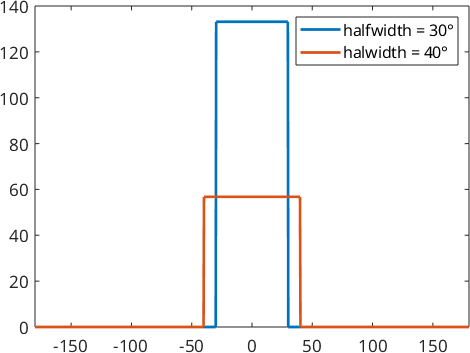We also take a look at the Fourier coefficients